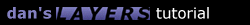2.2 Figure-8

I don't know how useful a figure-8 function will be, but as they say "the more the merrier". Most of the options are similar to before.

```function figure8() {
FigLayername = "layer1";  // name of the layer you want to move
FigRadius = 100;          // The length of one loop
FigMultiplier = 0.8;      // thickness multiplier, <1 makes thinner, >1 makes fatter
FigAnglestart = 0;        // starting angle
FigAngleinc = 3;          // angle incremenation, a larger number makes it go faster
FigDirection = "h";       // "h" for horizontal, "v" for vertical
FigRotationDir = 1;       // "1" for up/right first, "-1" for down/left first (depending on FigDirection)
FigSpeed = 20;            // repetition speed in milliseconds
FigAngle = FigAnglestart;
if (FigDirection == "h") {
}
if (FigDirection == "v") {
}
slidefigure8();
}

function slidefigure8() {
FigAngle += FigAngleinc;
if (FigDirection == "h") {
var x = FigLeft + FigRadius*Math.cos(FigAngle*Math.PI/180)*Math.abs(Math.cos(FigAngle*Math.PI/180));
var y = FigTop - FigRotationDir*FigMultiplier*FigRadius*Math.sin(FigAngle*Math.PI/180)*Math.cos(FigAngle*Math.PI/180);
}
if (FigDirection == "v") {
var x = FigLeft + FigRotationDir*FigMultiplier*FigRadius*Math.cos(FigAngle*Math.PI/180)*Math.sin(FigAngle*Math.PI/180);
var y = FigTop - FigRadius*Math.sin(FigAngle*Math.PI/180)*Math.abs(Math.sin(FigAngle*Math.PI/180));
}
document.layers[FigLayername].moveTo(x,y);
setTimeout("slidefigure8()",FigSpeed);
}
```1.1 Introduction 1.2 Overlapping 1.3 Nesting 1.4 Using JavaScript 2.1 Sliding Layers 2.2 Pre-Built Functions 2.3 Clipping Layers 2.4 Looping Animations 2.5 Changing Images 3.1 Mouse-Click Animation 3.2 Capturing Keystrokes 3.3 Drag and Drop 4.1 Making Demos 4.4 Problems 4.5 Screen Sizes Copyright © 1997 Dan Steinman. All rights reserved.